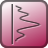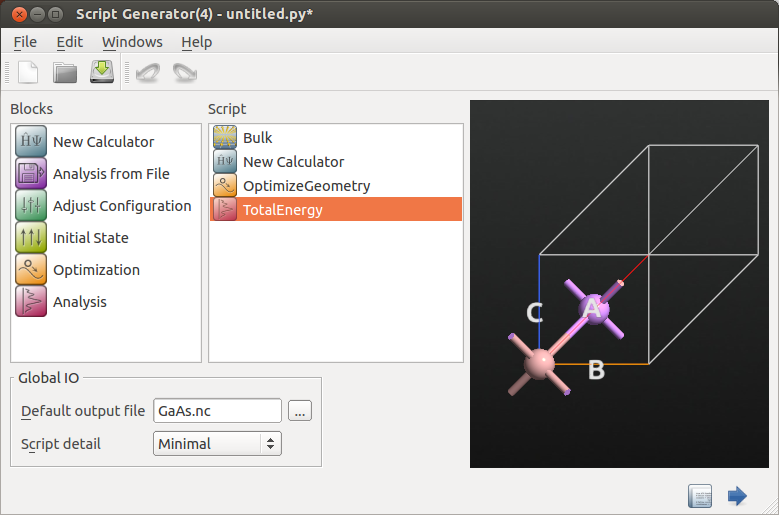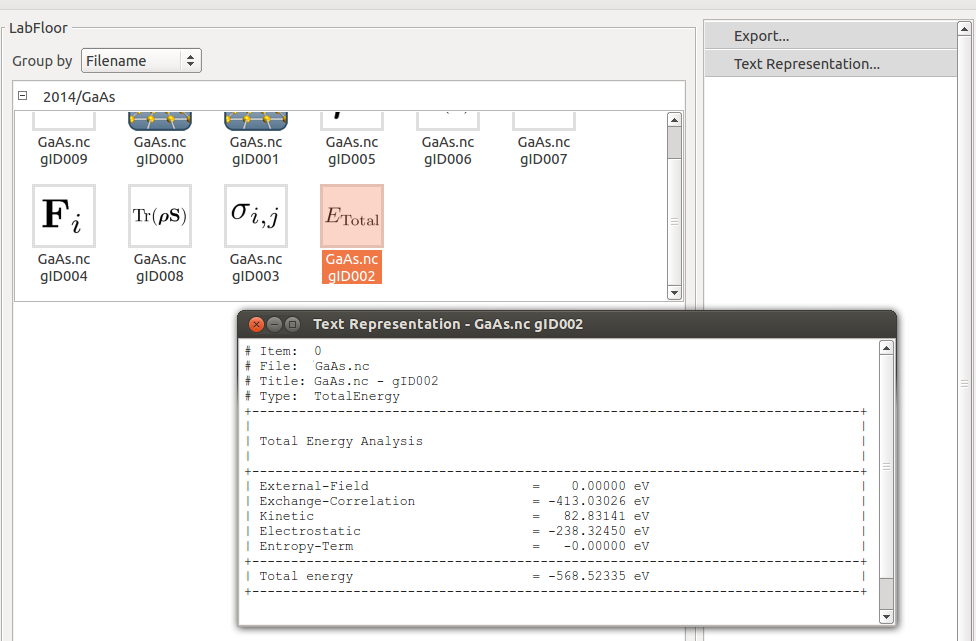# Calculation of Formation Energies¶

In this tutorial you will learn how to calculate cohesive and defect formation energies of different systems by total energy calculations. In particular, three examples are shown:

1. GaAs bulk;
2. Ga vacancy in GaAs;
3. Oxygen vacancy on a MgO surface.

## Formation energy calculations (or cohesive energy)¶

In ATK you can easily calculate the formation energy (or cohesive energy) of your system from conventional total energy calculations. In particular, you can calculate the formation energy from the total energy of your system and the total energy of its constituent parts:

(10)$E_{form} = E_{tot} – \Sigma_x E_{tot}(x).$

$$E_{form}$$ represents the energy required to dissociate the material into its individual components, $$x$$. You should pay particular attention when you choose the reference systems for your individual components, which could be bulk or gas phases.

Note

• $$E_{form}$$ is calculated from electronic structure calculations and does not take into account thermal and zero point energy terms (vibrational contributions).
• You may need well converged calculations (mesh cut-off and k-points).
• Remember to normalize the total energy you get by the number of atoms which compose your system.
• Pay attention to spin polarization. In particular if your reference system is a molecule with particular spin state, e.g. O2.

In order to calculate the total energy of your system, from theScripter add aGeometryOptimization block and aTotalEnergy analysis:Note

For a detailed explanation of each energy terms contained in the TotalEnergy analysis in ATK-DFT and ATK-Huckel forbulk anddevice configurations, see the notes in the TotalEnergy entry in the Reference Manual.

You can read the total energy of your system, in eV, in your log file and by analyzing the TotalEnergy object on the LabFloor using the Text Representation tool:## Cohesive energy of a bulk system¶

Let us take the simple example of a bulk system, GaAs, where you can take the bulk phases as reference systems for its individual components, Ga and As.

In this case you can calculate the formation energy of bulk GaAs from Ga and As bulk:

$E_{form}^\mathrm{GaAs} = E_{tot}^\mathrm{GaAs} - E_{tot}^\mathrm{Ga} / n_\mathrm{Ga} - E_{tot}^\mathrm{As} / n_\mathrm{As},$

where $$n_\mathrm{Ga}$$ and $$n_\mathrm{As}$$ are the number of atoms in the Ga and As bulk unit cells, respectively.

Results

With LDA and the FHI DoubleZetaPolarized basis set you will get $$E_{form}^\mathrm{GaAs}$$ = -0.6645 eV (exp: -0.73 eV ). The negative sign means that 0.6645 eV are gained when forming GaAs from Ga and As bulk.

Attention

The choice of the pseudopotentials and basis set can be crucial for formation energy calculations, and it very much depends on the elements included in your system.

In this particular case, the Ga element and its components are more sensitive to the pseudopotential and basis set definition.

In QuantumATK the HGH and OMX pseudopotentials are also available.

By using the HGH pseudopotentials (Tier 4 basis set) for the calculation of the formation energy of GaAs, you will get $$E_{form}^\mathrm{GaAs}$$ = -0.76 eV, in very good agreement with the reported experimental value .

Note

You can apply the same methods for other systems, such as molecular crystals. In this case, the terms $$\Sigma_x E_{tot}(x)$$ in the equation above corresponds to the total energies of the isolated molecules forming the crystal.

Attention

Be aware that you may need to apply the counterpoise correction to account to the basis set superposition error depending on the basis set. For more details please refer to the Grimme DFT-D2 and the BSSE counterpoise correction tutorial.

Note

You can also calculate the molecule formation energy from the total energy of your molecule and the total energy of the isolated atoms forming the molecule. Remember that especially in this case you should run spin-polarized calculations. Be also aware of DFT limitations in describing isolated species, e.g. O atom for the calculation of O2 formation energy .

## Defect formation energy calculations¶

By using the same approach you can calculate defect formation energies from total energy calculations.

### Ga vacancy in GaAs¶

Consider a GaAs crystal with a Ga vacancy. The defect formation energy can be calculated from:

(11)$E_{form} = E_{tot}^{\mathrm{Ga}_{1-x}\mathrm{As}} – E_{tot}^\mathrm{GaAs} + x \cdot E_{tot}^\mathrm{Ga},$

where $$E_{tot}^{\mathrm{Ga}_{1-x}\mathrm{As}}$$ is the total energy of GaAs containing $$x$$ gallium vacancies, $$E_{tot}^\mathrm{GaAs}$$ is the total energy of the perfect GaAs crystal, and $$E_{tot}^\mathrm{Ga}$$ is the total energy of bulk Ga.

Note

Besides the usual parameters to check for convergence you should also pay attention to the cell size which is directly related to the defect concentration.

Results

For LDA and FHI DoubleZetaPolarized basis set the ATK-DFT formation energy of a gallium vacancy in a 216 atoms GaAs unit cell is 3.15 eV (compared to 2.9 eV in ).

### Surface O vacancy on MgO(100)¶

Finally, let us take the example of an oxygen vacancy on a MgO(100) surface. In this case your reference system is either O2 in the gas phase or a single oxygen atom. The corresponding formation energies are reported in the table below for a 3x3 MgO(100) surface.

Formation energy (eV)
1/2 O2 O2
ATK-PBE 6.82 10.36
ATK-PBE - ghost atom 6.61 10.16
VASP PW91  6.32 9.48

Depending on the extension of your basis set you may need to include the BSSE correction as explained in the Grimme DFT-D2 and the BSSE counterpoise correction tutorial. In this case, the first term in Equation (11) is the total energy of the vacancy configuration with the atom that form the vacancy as ghost atom (ATK-PBE - ghost atom in the table), instead of actually removing them from the configuration.